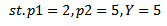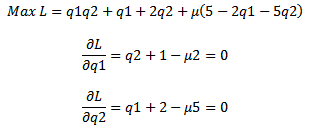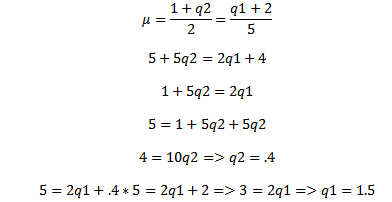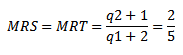Solving a Utility Max problem, a calculus and algabraic approach - FreeEconHelp.com, Learning Economics... Solved!

## 6/7/11Given this information, we can solve this problem in two different ways,
we can use calculus, or algebra.  For the calculus solution, we first have to set up the Lagrangian, which is a method used to account for the constraint in the optimization problem.  After this we get the first order conditions by taking partial derivatives with respect to each choice variable, our two different goods:Then we have to solve both equations for mu (µ) and set them equal to each other.  At this point we can then solve for 2q1, and plug into the constraint, use this to solve for q2, re-plug that number into the constraint and solve for q1, and you now have the optimal amounts of q1 and q2 to maximize utility subject to the budget constraint.For the algebraic approach, get the marginal rate of substitution, and the marginal rate of transformation and set them equal to each other,  then follow the above steps after getting rid of the mu.